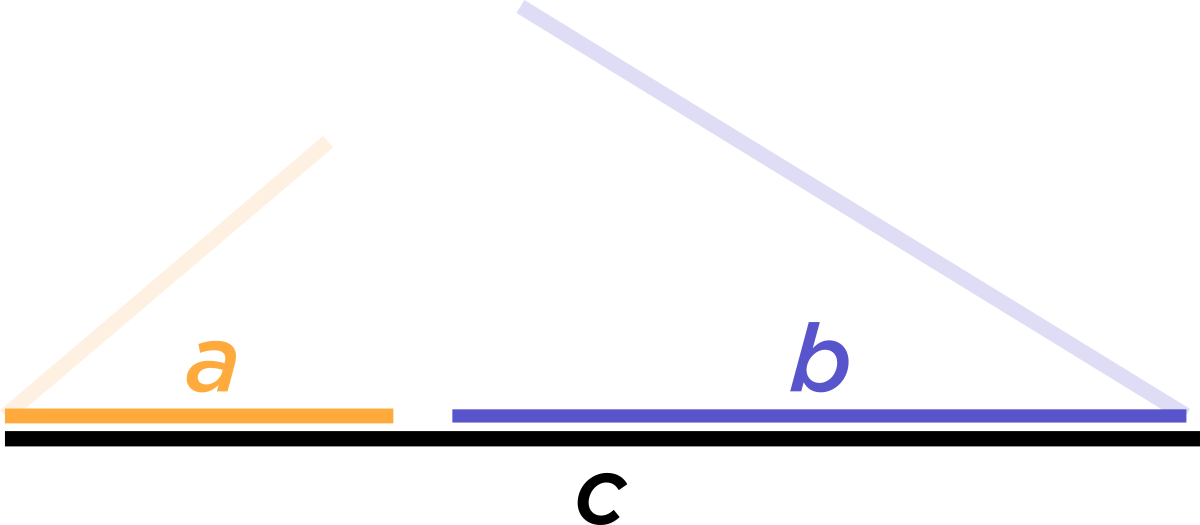# Factors of the Polynomial of Degree 4

• Why does the polynomial of degree 4 have the following factors?

$$a+b-c$$ $$a-b+c$$ $$-a+b+c$$ $$a+b+c$$

• a+b+c

Oh, wow, this is a nice question! (By the way, only the first three rows are factors of the $$\text{area}^2$$ formula.... not the $$a + b + c.$$ )

The fourth-degree polynomial that Prof. Loh is speaking about is the $$\text{(area)}^2$$ formula. He writes an ugly-looking expression down. But he's not looking at this ugly formula when he realizes that $$a + b - c, a - b + c,$$ and $$-a + b + c$$ are factors. He's just thinking about what the area of a triangle would be like if two of the sides added together were to equal the third side. (No algebra involved!!)You see, if $$a + b - c = 0,$$ then you can't make a triangle. You just get two straight lines that line up along the third line. And we call a "root" of a polynomial another expression that makes the polynomial $$0.$$

Like for example, consider $$x^2 + 5x + 6 = 0.$$ When $$x = 3,$$ then $$x^2 + 5x + 6 = 0,$$ so $$\textcolor{red}{x -3}$$ is a root of that polynomial. You can even factor the polynomial out as $$(\textcolor{red}{x-3})(x-2) = 0.$$

So we know that the area formula looks something like:

$$\text{ Area}^2 = (a + b -c)(a - b + c)(-a + b + c) \times \text{(some other stuff....)}.$$

Because when one of those terms (factors) equals $$0,$$ then the area equals $$0.$$

I hope this helped. Please let me know if there's something I can clarify for you! Thanks again for asking a great question!

• @debbie Ah, I see! Thank you for clarifying this, it definitely helped.

• @aaron-wang That's great!Have a!

• Can I have atoo

• @the-blade-dancerfor you (munch, munch)

• Hamburgers are cool